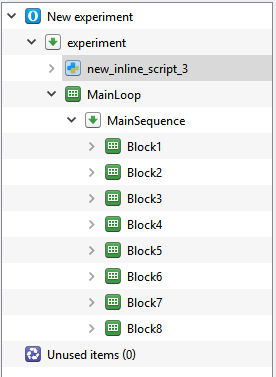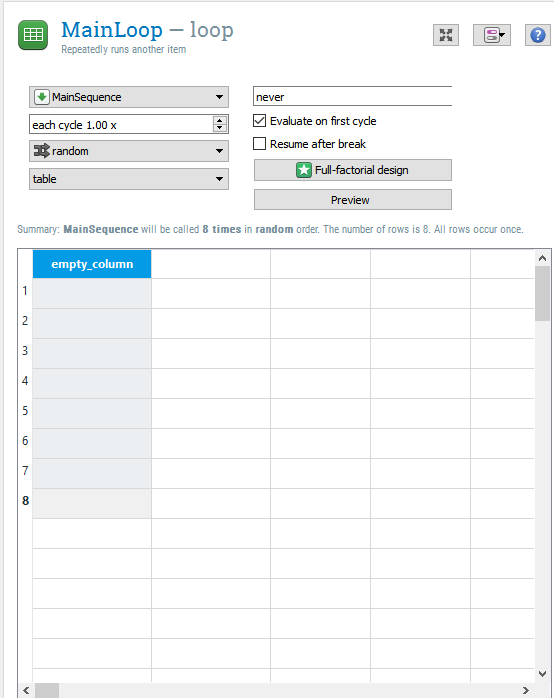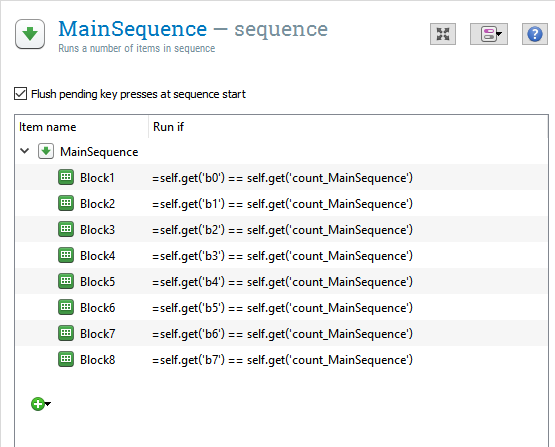#### Howdy, Stranger!

It looks like you're new here. If you want to get involved, click one of these buttons!

Supported by

# How to randomize blocks in OpenSesame (a solution not a question)

edited December 2016

Hello everyone,

I've had this problem where I wanted to randomize blocks of trials (i.e., randomize the presentation of the blocks, not the trials within a block). After some researching, I found Sebastian's youtube video on how to counterbalance blocks, and I found that extremely useful. However, if you would like to purely randomize blocks for each participant, I came up with a solution that I think anyone can do fairly simply. I am not a programmer, so if anyone sees something wrong or how to do this more simply, let me know!

1) The first step is to create an inline code at the beginning of the experiment (see the picture in step 2 to figure out where to place it. Mine is called "new_inline_script_3"):

``````from random import shuffle

#create a list of the number of blocks that you need. Always start at 0. Here I have eight blocks.

block_list = [0, 1, 2, 3, 4, 5, 6, 7]

#randomize the list that you just made
shuffle(block_list)

#create variables that will be used to help randomize later in your MainSequence. The number of variables depends on the number of blocks you have in your experiment (and should correspond to how many are in the block_list). So if you have only 3 blocks, you would use b0, b1 and b2.

b0 = block_list
b1 = block_list
b2 = block_list
b3 = block_list
b4 = block_list
b5 = block_list
b6 = block_list
b7 = block_list

#Make these variables part of the experiment

exp.set('b0',b0)
exp.set('b1',b1)
exp.set('b2',b2)
exp.set('b3',b3)
exp.set('b4',b4)
exp.set('b5',b5)
exp.set('b6',b6)
exp.set('b7',b7)
``````

2) Next set up your experiment hierarchically to include a Main Loop and Main Sequence. Call them MainLoop and MainSequence (if you call them something else, you will have to change the 'count_MainSequence' variable in step 4 to whatever you call your "MainSequence" sequence). Add the blocks within the Main Sequence.3) For the main loop, you need to have as many cycles as you have blocks. v3.1 is a bit different than previous versions in that you can't specify how many cycles you want through a drop down menu. But all you have to do is double click on cells in the first column to specify this.4) Click on the your Main Sequence and then add the following to the "run if" arguments next to each block:
block1 --> =self.get('b0) == self.get('count_MainSequence')
block2 --> self.get('b1') == self.get('count_MainSequence')"
etc...
Notice that the "b" variables start with 0. So Block 1 should be associated with b0

You can do this by going into the code itself:

``````    set flush_keyboard yes
set description "Runs a number of items in sequence"
run Block1 "=self.get('b0') == self.get('count_MainSequence')"
run Block2 "=self.get('b1') == self.get('count_MainSequence')"
run Block3 "=self.get('b2') == self.get('count_MainSequence')"
run Block4 "=self.get('b3') == self.get('count_MainSequence')"
run Block5 "=self.get('b4') == self.get('count_MainSequence')"
run Block6 "=self.get('b5') == self.get('count_MainSequence')"
run Block7 "=self.get('b6') == self.get('count_MainSequence')"
run Block8 "=self.get('b7') == self.get('count_MainSequence')"
``````

Or you can just click on the "run if" boxes and adding the code.That's it! Each time you run a participant, they should get a random order of each block. I hope this helps someone, and there is an easier way of doing this, let me know!